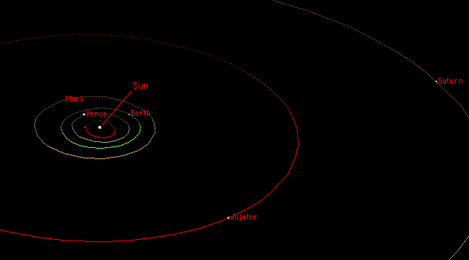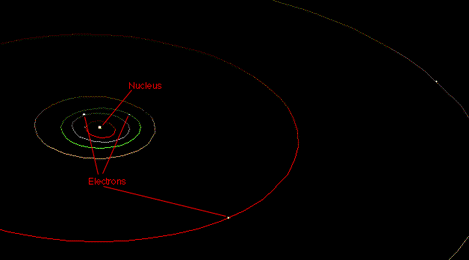# The Obvious Model

A little bit of mathematical detail is in order here. I've mentioned that the force between two electrically charged particles gets weaker as they get farther apart. In fact, the force gets weaker in proportion to the square of the distance between them. That is, if you take two charged particles some distance apart, and move one so that they're now three times as far apart, the force between them drops to 1/9 of its original strength. If you place them four times as far apart, the strength drops to 1/16 of what it was, and so on. This is sometimes referred to as an "inverse square" law. (Inverse, because as distance becomes greater, force becomes smaller.) This was discovered by a French physicist named Charles-Augustin de Coulomb.

By a happy coincidence (well, not exactly a coincidence) physicists already knew of another force that satisfies the same mathematical law. The numbers are a bit different, but it's an inverse square law, just the same. That force is gravity. Isaac Newton discovered that the gravitational force between celestial objects (the sun, planets, moons, etc.) obeys an inverse square law.

Now it's been known since the time of Johannes Kepler (before Newton) that the planets move in elliptical orbits around the sun. And Newton discovered that this is always the consequence of an inverse square force. All the planets move in ellipses around the sun, each planet's moons orbit the planet in elliptical orbits, and so on.Even comets, which may only come around once in a thousand years or more, move in very long, thin ellipses around the sun. And Coulomb discovered that the electric force is mathematically identical to the gravitational force.

So we're left with the inescapable conclusion that electrons must orbit the nucleus of an atom in elliptical paths.This, of course, is completely wrong.